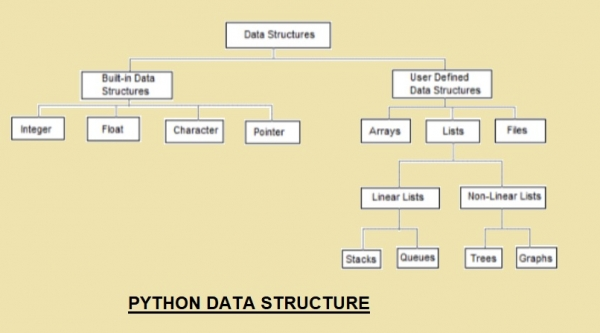# Why is python best suited for Competitive Coding

PythonServer Side ProgrammingProgramming

Competitive programming is generally referred to coding to make use of efficient algorithms using an appropriate data structure. They test the skills of programmers on many levels.

With the help of algorithms and data structures, you have to solve a hypothetical programming problem posed to you by applying different logics. You not only have to solve the problem but you have to come up with a very efficient solution, which is having a good time and space complexity.

Example of a problem statement for what is called competitive programming might be −

You are given a string s of length n consisting only of lowercase Latin letters.

A substring of a string is a contiguous subsequence of that string. So, string "forces" is a substring of string "codeforces", but string "coder" is not.

Your task is to calculate the number of ways to remove exactly one substring from this string in such a way that all remaining characters are equal (the number of distinct characters either zero or one).

It is guaranteed that there are at least two different characters in s.

Note that you can remove the whole string and it is correct. Also, note that you should remove at least one character.

To solve the above problem, you can use any programming language of your choice.

Now the question arises, why python for competitive coding?

## Speed

One of the reasons to choose python is that it radically cuts short the time you spend writing code, rather you spend time thinking about the logic that is needed for the question.

Because in this kind of competition time is the key, faster the programmer able to write the solution better it is. So, speed above I mean the speed with which a programmer write the solution, not the python language speed.

## Huge variety of libraries

Python comes with a vast variety of standard libraries and these libraries can be very well utilized in competitive programming.

Below is the list of other common reasons to choose python for competitive coding −

i.Common inbuilt functions:

• Python provides a large set of common functions like count, min, max, sorted etc. These inbuilt functions come very handy, allows coders to easily go ahead and skips writing code for these trivial procedures which often comes very useful. Also, python functions use the best algorithms for their functions. For example, the sorted() function uses the Timsort algorithm, which provides stable sorting at the worst case performance of O(nlogn). This is one of the best sorting algorithms which provides the best case run time of O(1) or constant run time.

## Code

#abs()
print(abs(-7))
#max()
print(max(2, 13, 4, 20))
#memoryview()
print(memoryview(bytes(9)))
#object()
o = object();print(type(o))
#pow()
print(pow(2,6))
#reversed
a = reversed([3, 13, 2, 1]); print(a)
#sorted()
print(sorted([9, 2, 4, 13, 7]))
#sum()
print(sum([2, 9, 12, 19]))
# type()
print (type([]))
print (type({}))
#zip()
print(set(zip([1,2],[3,4,5])))

ii. List Comprehensions

• Python provides one of the best list comprehensions. It allows us to write code which would generally take 5-20 lines in 1 line. With a List comprehension, you can have nested loops and conditions

## Code

# Iterating through a string Using List Comprehension
l_string = [ letter for letter in 'Tutorialspoint' ]
print( l_string)
#List Comprehensions vs Lambda functions
l_lambda = list(map(lambda x: x, 'Tutorialspoint'))
print(l_lambda)

## Output

['T', 'u', 't', 'o', 'r', 'i', 'a', 'l', 's', 'p', 'o', 'i', 'n', 't']
['T', 'u', 't', 'o', 'r', 'i', 'a', 'l', 's', 'p', 'o', 'i', 'n', 't']

• Python comes with a very extensive standard library, offering a wide range of facilities. These libraries come with several data structures which eliminate the need to implement them manually and also itertools which are a very important part of the library.
• For example, you want to generate all possible permutation of a list and store it in another single list, using list comprehension and permutation function from itertools.

## Python Standard Library

 Data types strings networking threads Operating System compression GUI arguments CGI complex numbers FTP cryptography testing multimedia databases CSV files calendar email XML serialization

iv.A vast variety of data structures

• Python provides a large set of the data structure to use in your coding including dictionary, sets, tuple, list and many others which comes as standard package.v.Ease of use

Python syntax is human readable and doing coding in python is very easy and fast. It basically reads like pseudocode.

>>> print("hello world!")
hello world!
>>> sum([3, 4, 3,5])
15
>>> max(3, 4, 5, 13,2)
13
>>> min(3, 4, 5, 13, 2)
2